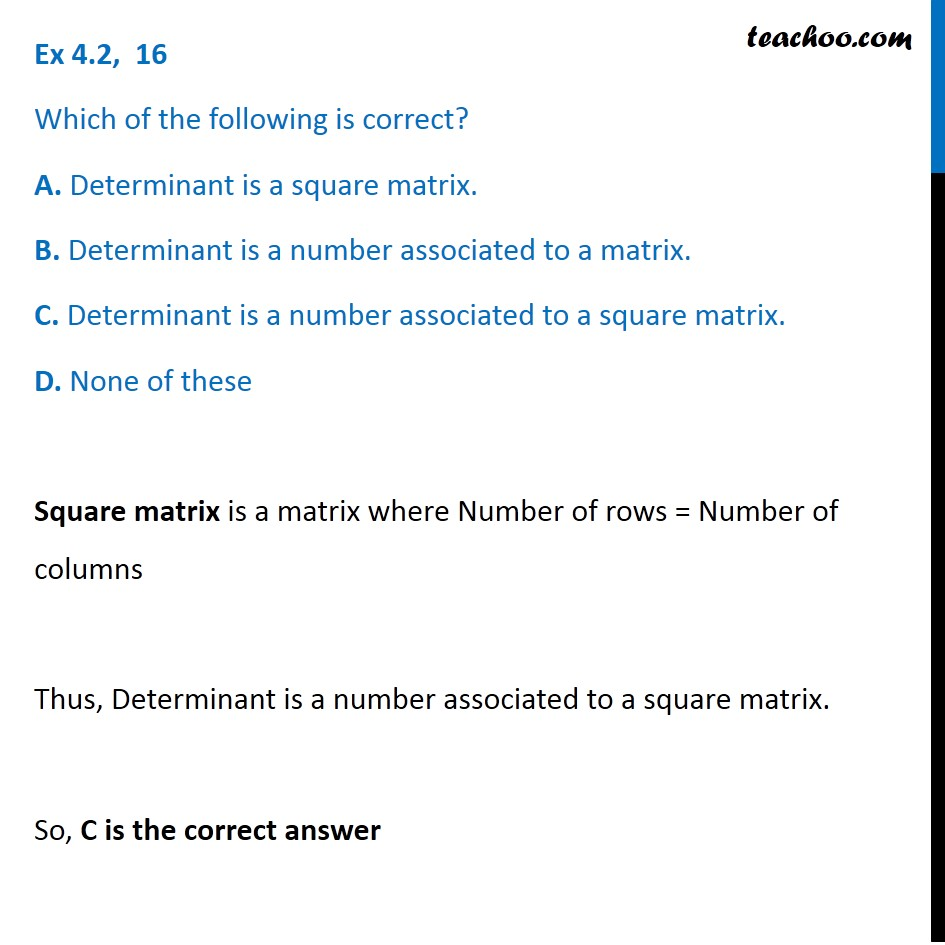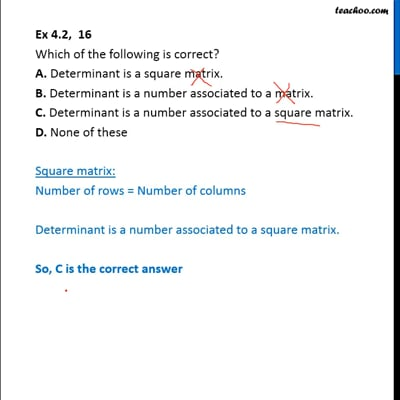Finding determinant of a 2x2 matrix

Chapter 4 Class 12 Determinants
Concept wiseThis video is only available for Teachoo black users

Introducing your new favourite teacher - Teachoo Black, at only ₹83 per month

### Transcript

Ex 4.2, 16 Which of the following is correct? A. Determinant is a square matrix. B. Determinant is a number associated to a matrix. C. Determinant is a number associated to a square matrix. D. None of these Square matrix is a matrix where Number of rows = Number of columns Thus, Determinant is a number associated to a square matrix. So, C is the correct answer